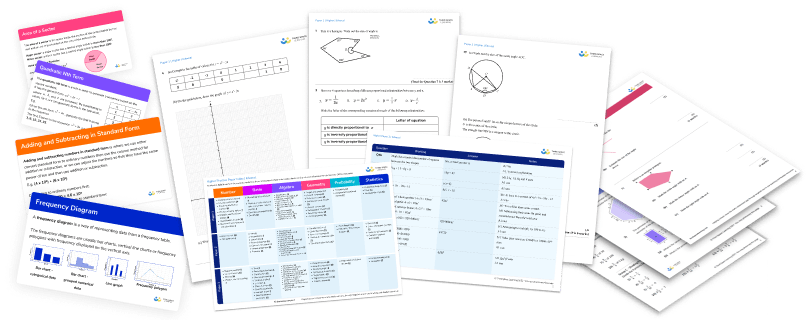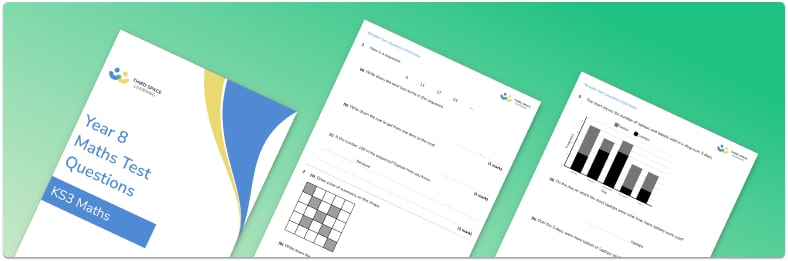Teaching support from the UK’s largest provider of in-school maths tuition.one to one lessonsschools supported

The best start to KS3

Scalable, affordable online support for Year 7 students from maths and pedagogy experts

View Year 7 Programme

FREE KS3 maths resources

Here you can find all of our free KS3 resources; explore the collections or navigate to each section to filter resources by topicExplore all resources
Key Stage 3

The best start to KS3

Scalable, affordable online support for Year 7 students from maths and pedagogy experts

View Year 7 Programme

# Free Year 8 Maths Test With Answers and Mark Scheme

This year 8 maths test is suitable for the majority of year 8 students and contains questions on a wide variety of topics. The test includes both procedural questions and applied problems.

This test is designed to be taken at the end of year 8. If you’re looking for a test for the start of year 8 we recommend you download the free year 7 maths test instead as it will provide a much fairer assessment of what students know.

### What topics are likely to be covered in Year 8 maths

The maths that students learn in year 8 builds on the maths that they will have learnt in year 7. They will cover topics from across all of the 6 areas of maths; number, ratio and proportion, algebra, geometry, statistics and probability.

By year 8, students are more familiar with the concept of algebra meaning this can be studied in a more formal way and also used to solve simple problems. Topics that may be introduced for the first time in year 8 include prime factors, HCF and LCM, indices, inequalities, area of a trapezium and a circle, angles in polygons and  Pythagoras theorem.

Check out our comprehensive revision guides and maths worksheets for these topics here:Year 8 Maths Test

Download a free end of term Year 8 maths test with 16 practice maths questionsDownload Free Now!

### Year 8 maths questions

Students in year 8 are expected to answer a variety of questions. Some questions will be procedural and will focus on learning and practising new methods. Other questions will ask students to apply their knowledge to solve problems. By year 8, most students should be comfortable with areas of numeracy such as mental and written methods, fractions, decimals and percentages and can be challenged to solve multi-step problems in these areas.

Our year 8 test contains 16 questions of increasing difficulty. The test begins with questions accessible to the vast majority of year 8 students and the questions get progressively more challenging. The maths topics covered in the test are listed in the table below:

The total number of marks available for this test paper is 50 and each question is worth between 1 and 3 marks. There are a range of question styles, including procedural questions, reasoning questions and multi-step applied questions. The mix of questions is aimed at creating familiarity with the style of a GCSE maths exam paper.

### How to use this Year 8 maths test

Our year 8 maths test is designed to be used as an end of year test. The test is one hour long, enabling it to be sat within a lesson.

Students should be encouraged to show all of their working just as for any practice test. Students may use a calculator during the test.

Once students have finished, you may to mark these yourself or distribute the answers and mark scheme to students to peer or self assess. You can also give effective feedback without adding to your workload by using whole class feedback – we’ve even got a FREE downloadable template for you.

### Mark scheme and grade boundaries

There is a mark scheme provided with the test, including a breakdown of marks when a question is worth more than one mark.

### Year 8 Maths test

Questions follow. Don’t forget to download the free Year 8 maths test for the answers and mark scheme to accompany these questions.

Looking for the answers? Download the free year 8 maths test as a pdf for the answers and mark scheme to accompany these questions.

### What maths should a Year 8 know?

By the end of year 8, students will have covered a good amount of the KS3 maths curriculum. They will have gained a broad understanding of many topics across the six main areas of maths; number, ratio and proportion, algebra, geometry, statistics and probability.

These include:

• Number: fractions, decimals, percentages, place value, negative numbers, factors and multiples, rounding, order of operations
• Algebra: manipulating algebraic expressions, expanding and factorising, solving linear equations, using formulae, sequences, straight line graphs, inequalities
• Ratio, proportion and rates of change: ratio, direct proportion, conversion rates
• Geometry and measures: area and perimeter, surface area and volume of 3D shapes, angles, parallel lines, properties of polygons, transformations, speed/distance/time, construction and loci, bearings, Pythagoras theorem
• Probability: theoretical probability, experimental probability, sample space diagrams, Venn diagrams
• Statistics: data collection, pie charts, scatter diagrams, averages

It is worth noting that different schools cover the curriculum in different orders and there is no set list of what a student should know by the end of year 8.

### How do I prepare for Year 8?

Year 8 maths builds on the maths done in year 7. You can prepare for year 8 maths by revising topics covered in years 7 and ensuring you are confident with as many of these as possible. Some good resources to help you with this are:

### What level is Year 8 maths?

By the end of year 8 most students will be working between GCSE grades 1 and 4. Different schools use different types of levels for key stage 3 maths so it is best to check how your school assigns levels to your work.

Year 8 work builds on work done in year 7, with the introduction of some new topics and more challenging problem solving questions.

#### More support for Year 8

If there is a particular topic or area of study that you would like more ideas or support with, check out the GCSE maths revision pages from Third Space Learning, many of which are also relevant and useful to Year 8 maths students.

Do you have students who need extra support in maths?
Every week Third Space Learning’s maths specialist tutors support thousands of students across hundreds of schools with weekly online 1-to-1 lessons and maths interventions designed to plug gaps and boost progress.

Since 2013 we’ve helped over 100,000 primary and secondary students become more confident, able mathematicians. Find out more about our GCSE Maths Revision Programme or request a personalised quote for your school to speak to us about your school’s needs and how we can help.

Personalised one to one maths tutoring for Year 7 students designed to help ease the transition to KS3.##### Beki Christian
Maths Teacher and Author
Beki has 11 years experience teaching secondary Maths. She is currently working as a Maths tutor as well as writing resources and blog posts for Third Space Learning.

Related Articles

x#### Year 8 Maths Test

Download a free end of term Year 8 maths test with 16 practice maths questions

#### Year 8 Maths TestDownload a free end of term Year 8 maths test with 16 practice maths questions